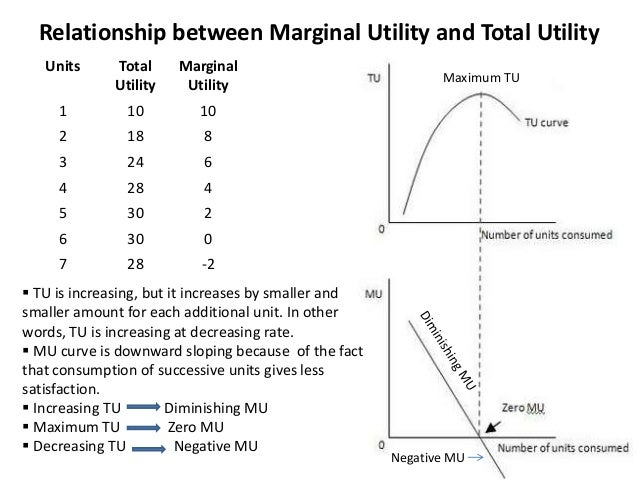Home / Economics / Total utility (TU) and Marginal Utility (MU)

# Total utility (TU) and Marginal Utility (MU)

#### Total utility (TU) and Marginal Utility (MU):

Total Utility (TU) Definition and Explanation

“Total utility is the total is the total satisfaction obtained from all units of a particular commodity consumed over a period of time”.

For example, a person consumes eggs and gains 50 units of total utility. This total utility is the sum of Utilities from the successive units (30 units from the first egg, 15 units from the second and 5 units from the third egg.

Summing up total utility is the amount of satisfaction (utilty) obtained from consuming a particular quantity of a god or service within a given time period. It is the sum of marginal utilities of each successive unit of consumption.

Formula: TU x =∑MUX

Marginal Utility (MU):

Marginal utility means an additional or incremental utility. Marginal utility is the change in the total utility is the change in consumption of the commodity within a given period of time”.

For example: when a person increases the consumption of eggs from one egg to two eggs, the total utility increases from 30 units to 45 units. The marginal utility here be the 15 unites of the 2nd egg consumed.

Marginal utility, thus, can also be described as difference between total utility derived from one level of consumption and total utility derived from another level of consumption.

Formula:

Or, in other word MUn = TUn –TUn-1

Page 105

Relationship between Total Utility (tU) and Marginal Utility (MU):

It may be noted that as a person consumes more and more units of a commodity, the marginal utility of the additional units begins to diminish but the total utility goes on increasing at a diminishing rate.

When the marginal utility comes to zero or we say the point of satiety is reached, the total utility is the Maximus. If consumption is increased further from this point of satiety, the marginal utility becomes negative and total utility begging to diminish.

The relationship between total and marginal utility is now explained with the help of following schedule and a graph.

 Units of apples Consumed Daily Total Utility in units Per Day Marginal Utility in Units Per Day 1 2 3 4 5 6 7 11 13 14 14 15 7 4(11-7) 2(13-11) 1(14-13) 0(14-14) -1(13-14)

The above table shows that when a person consumes no apples, he gets no satisfaction. His total utility is zero. In case he consumes one apple a day, he gains seven units of satisfaction. His total utility is 7 and his marginal utility is also 7.

In case he consumes second apple, he gains extra 4 units (MU). Thus given him a total utility of 11 units of 11 units from two apples.

Marginal utility has gone down from 7 units to 4 units because he has a less craving for the second apple. Same is the case with the consumption of third apple. The marginal utility has now fallen to 2 units while the total utility of three apples has increased to 13 units ( 7 + 4 + 2 ). In case the consumer takes fifth apple, his marginal utility falls to zero units and if he consumes sixth apple also, the total showing total utility and marginal utility is plotted in figure below:

Diagram/Curve:1. The total utility curves starts at the origin as zero consumption of apples yield zero utility.
2. The TU curve reaches at its maximum or a peak of M when MU is zero.
3. The MU curve falls through the graph. A special point occurs when the consumer consumes fifth apples. He gains no marginal utility from it. After this point marginal utility becomes negative.